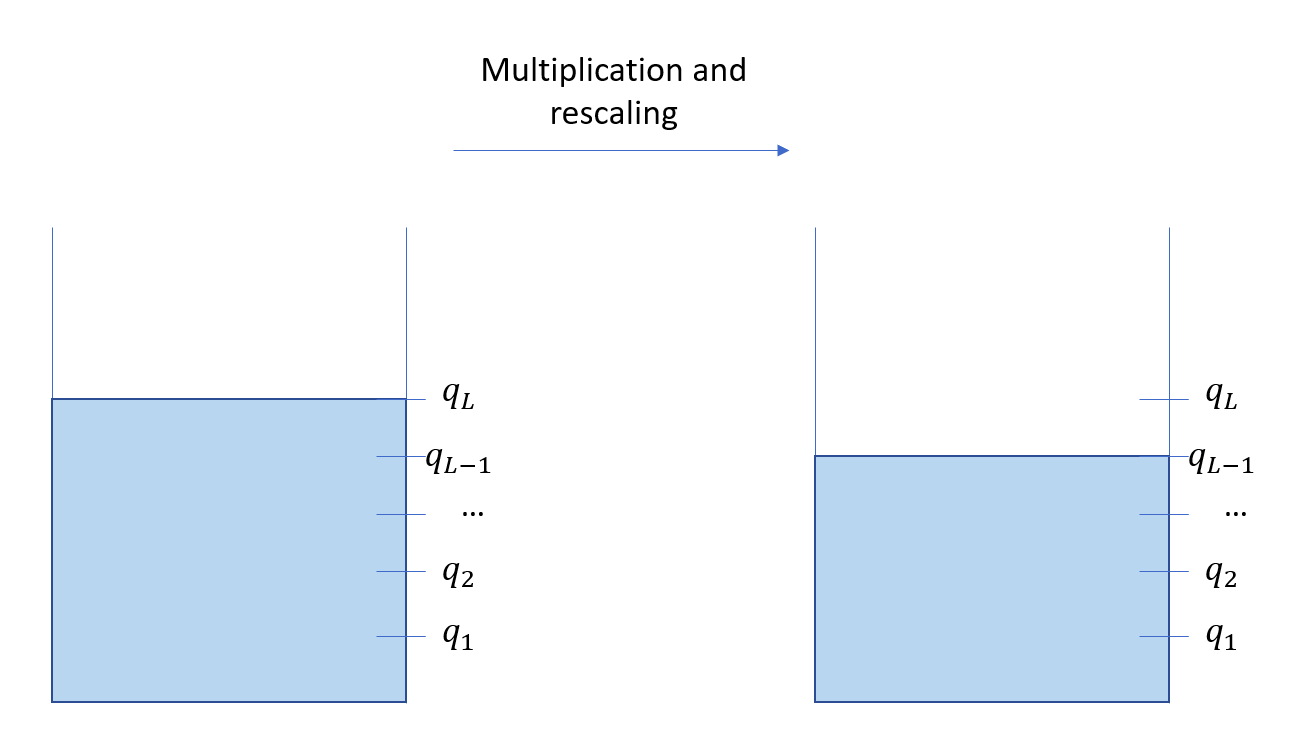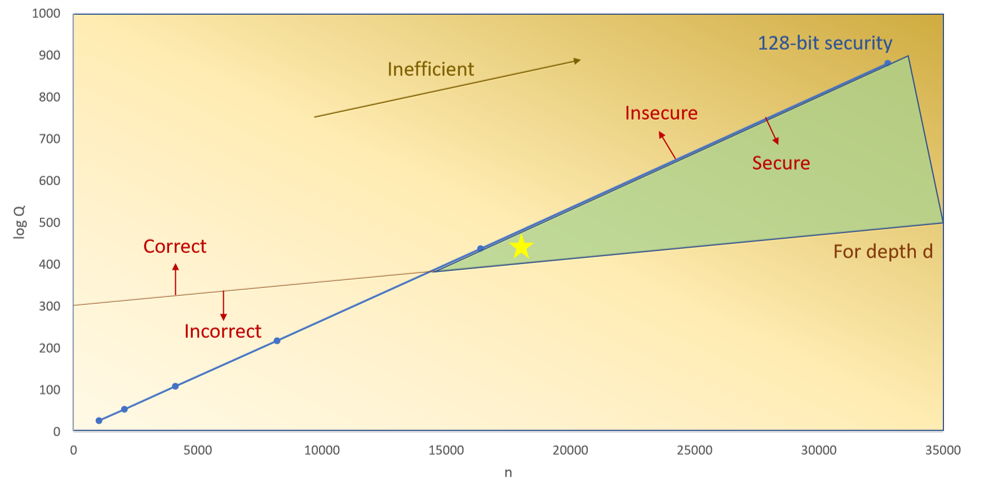# CKKS Part5: CKKS的重缩放

## 模数链的高级视图1、重缩放和噪音管理可以被视为管理一个油箱，你从一个初始预算（油量）开始，使用（做乘法）后预算（油量）将会减少，如果汽油用完了，你就什么都做不了。
2、你需要提前知道你将进行多少次乘法，这将决定油箱的大小，这将影响你将使用的多项式次数的大小。

## 普通做法$R\mbox{S}_{l->l-1}\left( c \right)=\left\lfloor \frac{q_{l-1}}{q_{l}}.c \right\rfloor\left( \mbox{mod}\; q_{l-1} \right)=\left\lfloor \Delta ^{-1}.c \right\rfloor\left( \mbox{mod}\; q_{l-1} \right)$

1、一旦我们解密了两个密文c，c′的乘积，以及对应底层明文值$$\Delta .z,\Delta .z'$$，应用重缩放后，我们有$$\Delta .z.z'$$，因此，只要我们在每次乘法后重缩放，在整个计算过程中，缩放因子保持不变。
2、噪声被减少了，因为我们既分割底层的明文值，也分割了解密时的噪声，如果你记得清楚的话，它的形式是μ+e，（此时解密，再舍入得到的u不是原先的明文值）。因此，重缩放也可以达到降噪的目的。

1、做乘法：$$c_{mult}=\mbox{C}_{Mult}\left( c,c' \right)=\left( d_{0},d_{1},d_{2} \right)$$
2、重线性化：$$c_{\mbox{re}lin}=\mbox{Re}lin\left( \left( d_{0},d_{1},d_{2} \right),evk \right)$$
3、重缩放：$$c_{rs}=R\mbox{S}_{l->l-1}\left( c \right)$$

## CRT，中国剩余定理

posted @ 2022-02-07 09:36  PamShao  阅读(535)  评论(2编辑  收藏  举报